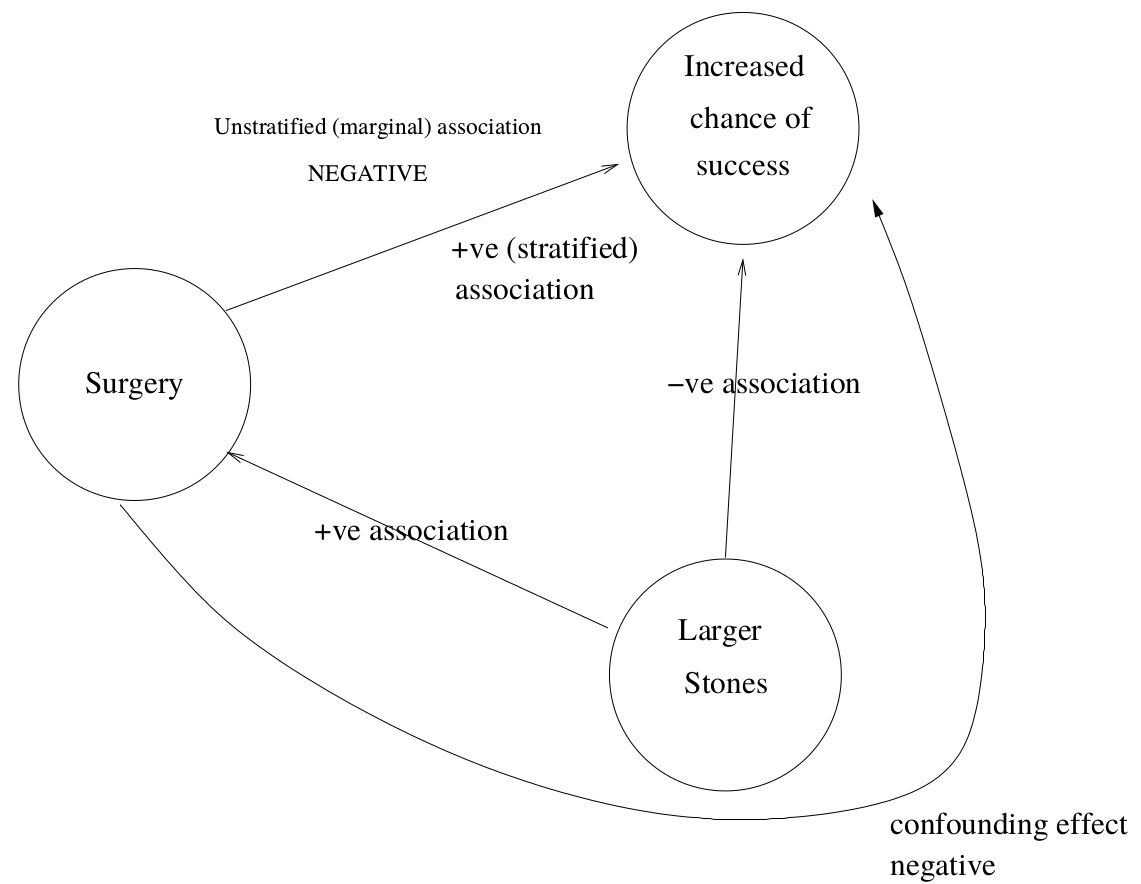# 第 49 章 混雜的調整，交互作用，和模型的可壓縮性

• 與關心的預測變量相關 (i.e. $$\delta_1 \neq 0$$)；
• 與因變量相關 (當關心的預測變量不變時，$$\beta_2\neq0$$ )；
• 不在預測變量和因變量的因果關係 (如果有的話) 中作媒介。Not be on the causal pathway between the predictor of interest and the dependent variable.

< 2cm Diameter
>= 2cm Diameter
Group Surgery Lithotripsy Surgery Lithotripsy
Success 81.00 234 192.00 55
Failure 6.00 36 71.00 25
Total 87.00 270 263.00 80
Odds Ratios 2.08 NA 1.23 NA

$$<2$$ cm Diameter
$$\geqslant2$$ cm Diameter
Group Surgery Lithotripsy Surgery Lithotripsy
Success 81 234 192 55
Failure 6 36 71 25
Total 87 270 263 80
Odds Ratios 2.08 1.23

Outcome Surgery Lithotripsy
Success 273 (78%) 289 (83%)
Failure 77 61
Total 350 350
Odds ratio 0.75

Outcome Surgery Lithotripsy
Success 273 (78%) 289 (83%)
Failure 77 61
Total 350 350
Odds ratio 0.75

Size of the Stone Surgery Lithotripsy
$$< 2$$ cm 87 (33%) 270 (77%)
$$\geqslant 2$$ cm 263 80
Total 350 350

Outcome $$< 2$$ cm $$\geqslant 2$$ cm
Success 234 (87%) 55 (69%)
Failure 36 25
Total 370 80

Outcome $$< 2$$ cm $$\geqslant 2$$ cm
Success 81 (93%) 192 (73%)
Failure 6 71
Total 87 263## 49.1 混雜因素的調整

Group
$$X=0$$ $$X=1$$
$$D=0$$ $$X=0$$ $$n_{00}$$ $$n_{10}$$
$$X=1$$ $$n_{01}$$ $$n_{10}$$

### 49.1.1 Woolf 法估算合併比值比

$\text{Var}(\text{log}\hat\Psi_i) \approx \frac{1}{a_i} + \frac{1}{b_i} + \frac{1}{c_i} + \frac{1}{d_i} = \frac{1}{w_i}$

$\text{log}\hat\Psi_w = \frac{\sum w_i\text{log}\hat\Psi_i}{\sum w_i}$

$\text{Var}(\text{log}\hat\Psi_w) = \frac{1}{\sum w_i}$

\begin{aligned} \hat\Psi_1 = 2.08 ;&\; \hat\Psi_2 = 1.23 \\ \text{Var}(\text{log}\hat\Psi_1) = \frac{1}{81} & + \frac{1}{234} + \frac{1}{6} + \frac{1}{36} = 0.2111 \\ \text{Var}(\text{log}\hat\Psi_2) = \frac{1}{192} & + \frac{1}{55} + \frac{1}{71} + \frac{1}{25} = 0.0775 \\ w_1 = \frac{1}{\text{Var}(\text{log}\hat\Psi_1)} = 4.74 ; \;& w_2 = \frac{1}{\text{Var}(\text{log}\hat\Psi_2)} = 12.91 \\ \text{log}\hat\Psi_w = & \frac{4.74\times\text{log(2.08)} + 12.91\times\text{log(1.23)}}{4.74 + 12.91} \\ = & 0.3481 \\ \Rightarrow \hat\Psi_w =& e^{0.3481} = 1.42\\ \text{Var}(\hat\Psi_w) =& \frac{1}{4.74+12.91} = 0.0567 \\ \Rightarrow 95\% \text{ CI} = & e^{0.3481 \pm 1.96\times\sqrt{0.0567}} \\ = & (0.89, 2.26) \end{aligned}

Woolf 的計算調整後的合併比值比的方法是在線性迴歸和廣義線性迴歸被發現之前誕生的，但是其想法之精妙，確實令人感嘆。可惜其最大的缺陷是無法用這樣的方法進行連續型變量的調整，也很難同時進行多個變量的調整，所以現在這一算法已經逐漸被淘汰。現在我們有了廣義線性迴歸模型這一更強大的工具，只要把變量加入廣義線性模型進行調整就可以計算曾經難以計算和擴展的調整後的合併比值比。從下面的代碼計算獲得的調整後比值比 $$1.43 (0.91, 2.34)$$ 也可以看出，Woolf 方法的計算結果也是足夠令人滿意的。

size <- c("< 2cm", "< 2cm", ">= 2cm", ">= 2cm")
treatment <- c("Surgery","Lithotripsy","Surgery","Lithotripsy")
n <- c(87, 270, 263, 80)
Success <- c(81, 234, 192, 55)
Stone <- data.frame(size, treatment, n, Success)
ModelStone <- glm(cbind(Success, n - Success) ~ treatment + size, family = binomial(link = logit), data = Stone)
summary(ModelStone)
##
## Call:
## glm(formula = cbind(Success, n - Success) ~ treatment + size,
##     family = binomial(link = logit), data = Stone)
##
## Deviance Residuals:
##        1         2         3         4
##  0.76357  -0.35881  -0.27563   0.46948
##
## Coefficients:
##                  Estimate Std. Error z value  Pr(>|z|)
## (Intercept)       1.93655    0.17045 11.3614 < 2.2e-16 ***
## treatmentSurgery  0.35723    0.22908  1.5594    0.1189
## size>= 2cm       -1.26057    0.23900 -5.2742 1.333e-07 ***
## ---
## Signif. codes:  0 '***' 0.001 '**' 0.01 '*' 0.05 '.' 0.1 ' ' 1
##
## (Dispersion parameter for binomial family taken to be 1)
##
##     Null deviance: 33.12395  on 3  degrees of freedom
## Residual deviance:  1.00816  on 1  degrees of freedom
## AIC: 26.3554
##
## Number of Fisher Scoring iterations: 3
epiDisplay::logistic.display(ModelStone)
##
## Logistic regression predicting cbind(Success, n - Success)
##
##                                   crude OR(95%CI)   adj. OR(95%CI)    P(Wald's test) P(LR-test)
## treatment: Surgery vs Lithotripsy 0.75 (0.51,1.09)  1.43 (0.91,2.24)  0.119          < 0.001
##
## size: >= 2cm vs < 2cm             0.34 (0.23,0.51)  0.28 (0.18,0.45)  < 0.001        < 0.001
##
## Log-likelihood = -10.1777
## No. of observations = 4
## AIC value = 26.3554

## 49.2 交互作用

ModelStone2 <- glm(cbind(Success, n - Success) ~ treatment*size, family = binomial(link = logit), data = Stone)
summary(ModelStone2)
##
## Call:
## glm(formula = cbind(Success, n - Success) ~ treatment * size,
##     family = binomial(link = logit), data = Stone)
##
## Deviance Residuals:
##   0  0  0  0
##
## Coefficients:
##                             Estimate Std. Error z value  Pr(>|z|)
## (Intercept)                  1.87180    0.17903 10.4553 < 2.2e-16 ***
## treatmentSurgery             0.73089    0.45942  1.5909 0.1116310
## size>= 2cm                  -1.08334    0.30039 -3.6065 0.0003104 ***
## treatmentSurgery:size>= 2cm -0.52453    0.53716 -0.9765 0.3288211
## ---
## Signif. codes:  0 '***' 0.001 '**' 0.01 '*' 0.05 '.' 0.1 ' ' 1
##
## (Dispersion parameter for binomial family taken to be 1)
##
##     Null deviance: 3.31239e+01  on 3  degrees of freedom
## Residual deviance: 3.66374e-14  on 0  degrees of freedom
## AIC: 27.3472
##
## Number of Fisher Scoring iterations: 3
epiDisplay::logistic.display(ModelStone2)
##
## Logistic regression predicting cbind(Success, n - Success)
##
##                                   crude OR(95%CI)   adj. OR(95%CI)    P(Wald's test) P(LR-test)
## treatment: Surgery vs Lithotripsy 0.75 (0.51,1.09)  2.08 (0.84,5.11)  0.112          < 0.001
##
## size: >= 2cm vs < 2cm             0.34 (0.23,0.51)  0.34 (0.19,0.61)  < 0.001        < 0.001
##
## treatmentSurgery:size>= 2cm       -                 0.59 (0.21,1.7)   0.329          < 0.001
##
## Log-likelihood = -9.6736
## No. of observations = 4
## AIC value = 27.3472

## 49.3 可壓縮性 collapsibility

### 49.3.1 線性迴歸的可壓縮性

$$Y$$ 標記結果變量，$$X$$ 標記暴露變量，$$Z$$ 則標記我們想要調整的莫個混雜因子：

$Y = \alpha + \beta_X X + \beta_Z Z + \varepsilon, \text{ where } \varepsilon \sim N(0, \sigma^2)$

$E(Y | X) = \alpha + \beta_X X + \beta_Z E(Z|X)$

$E(Y|X) = \alpha + \beta_Z \mu_Z + \beta_X X$

### 49.3.2 邏輯鏈接方程時的不可壓縮性

Strata 1
Strata 2
Outcome Exposure $$+$$ Exposure $$-$$ Exposure $$+$$ Exposure $$-$$
Success 90 50 50 10
Failure 10 50 50 90
Total 100 100 100 100
Odds Ratios 9 9

Outcome Exposure $$+$$ Exposure $$-$$
Success 140 60
Failure 60 140
Total 200 200
Odds ratio 5.4

## 49.4 交互作用對尺度的依賴性

GLM 模型中的交互作用檢驗，對選用的尺度 (比值比 OR，還是危險度比 RR) 依賴性極高。用模型可壓縮性的數據例子也可以說明交互作用對尺度的依賴性。上文書說到，兩個分層中的比值比都是 9，該分層變量既沒有交互作用，也不是混淆因子 (當使用比值比的時候)。如果我們改用危險度比 (risk ratio, RR)，在分類變量的第一層 (Strata 1) 中，暴露的危險度比是 $$\frac{90/100}{50/100} = 1.8$$；分類變量的第二層 (Strata 2) 中，暴露的危險度比是 $$\frac{50/100}{10/100} = 5$$。所以使用危險度比作爲評價指標的時候，被調整的分類變量就突然搖身一變變成了有交互作用的因子。這裏，我們用數據，證明了交互作用的存在與否，對尺度的選用依賴性極高。這就導致我們在描述一個變量是否對我們關心的暴露和結果之間的關係有交互作用時，必須明確指出所使用的是比值比，還是危險度比進行的交互作用評價。